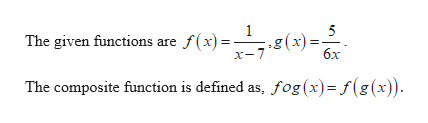# For the given functions f and g, find the indicated composition.f(x)=1/x-7          g(x)=5/6x(f o g)(x)

Question
7 views

For the given functions f and g, find the indicated composition.

f(x)=1/x-7          g(x)=5/6x

(f o g)(x)

check_circle

Step 1help_outlineImage TranscriptioncloseThe given functions are f(x)= 1 5 .g(x). бх The composite function is defined as, fog(x)=f(g(x)) fullscreen

### Want to see the full answer?

See Solution

#### Want to see this answer and more?

Solutions are written by subject experts who are available 24/7. Questions are typically answered within 1 hour.*

See Solution
*Response times may vary by subject and question.
Tagged in

### Algebra